## General Question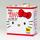# How do I get OpenOffice Calc to format 69% as 69% instead of 6900%?

Asked by bootonthroat (344) June 30th, 2010

Imagine you have a column APR and in it you have the value “3.5”. How do I display a percent sign after the 3.5? I can only figure out how to display numbers which are NOT percentages with a % such as 0.035. Notice 0.035 is NOT a percentage but will display as 3.5% when converted to one. I already have the numbers as percentages. It doesn’t seem unreasonable that I should want to display a % sign after a percentage but it does appear impossible as far as I can determine.

Observing members: 0Composing members: 0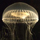The problem here is one of mathematics I think. 0.035 is in fact 3.5% (that is, it’s 3.5 hundredths of 1. Similarly, 69 is 6900 hundredths of one. These systems (excel is the same), are trained to recognize decimals and their equivalent %ages as the same – therefore .5 and 50% are the same thing. If you have a column of numbers that are the % you want to display, make another column dividing the first by 100, then make that column into %ages. 69/100 = .69 = 69%.

kfingerman (992)“Great Answer” (2) Flag as…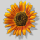In excel, you would go to Format>Cells>Numbers, select Percentage and enter the number of places after the decimal that you want displayed. When you go to enter a number in a cell, it displays with the percentage sign.

I don’t have OpenOffice, but this would seem to be pretty standard functionality.

PandoraBoxx (18011)“Great Answer” (1) Flag as…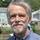I have OpenOffice and Excel. They should behave the same. @PandoraBoxx‘s fix should work.

ETpro (34543)“Great Answer” (0) Flag as…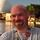@kfingerman is 100% correct. Your problem is that you’re asking OpenOffice to be mathematically incorrect. Fix your column’s values to be a percentage by dividing by 100, and it will display the way you want.

MrItty (17381)“Great Answer” (0) Flag as…@MrItty
“Fix your column’s values to be a percentage by dividing by 100” <== INCORRECT My column’s values ARE percentages. If I divide these by 100 I will then have decimals!

@kfingerman @ETpro (especially @ETpro because I think you have the best chance of grasping this).

“3.5% is in fact 0.035”
and
”\$8.50 is in fact 850¢”

Now let us move on to something we can also agree on:
If you ask, how many dollars do we have the answer is not equal to how many cents do we have. In fact, the answers are off by 100 fold: (8.5 <> 850). If we ask how many percent do we have the answer is also 100 times greater than simply asking for the decimal value (3.5 <> 0.035).

Therefore:
If you have a value X it could represent cents, percentage points, dollars, or a decimal (or many other things).

This gives us 4 numbers we can store in a cell from our example each representing a different type of quantity:
850 (A: representing pennies)
8.5 (B: representing dollars)
3.5 (C: representing a %)
0.035 (D: representing a decimal)

Please note that the numbers I have in my column represent a %, not a decimal I wish to then have the spreadsheet “help” me display as a percent. Since these numbers represent a percent all of my formulas multiply and divide this number by 100 when the formula calls for a decimal value.

Back to the question:
Is there any way to tell oocalc that these numbers represent a percent and NOT a decimal value? I begin to suspect the answer is NO but it would not surprise me if someone had a “units” plugin or some other “trick” to let oocalc know the value IS A PERCENT and not a decimal I wish to format and display as a percent. Even if there is no way to tell oocalc that the value is a percent is there really no display formatting option allowing me to display it as one?

Do others not have great demand for a way to assign a unit to a cell? To me a numerical cell should contain a vector of numbers (in case the value is complex, etc but normally containing only a single element). It should contain a base unit (such as m/s). It should contain a display as unit (such as mi/hr) and then it should contain some logic for rounding, scientific notion, number after the decimal, etc.

bootonthroat (344)“Great Answer” (0) Flag as…@bootonthroat you need to go back to 5th grade mathematics class, because this statement is simply wrong: “My column’s values ARE percentages. If I divide these by 100 I will then have decimals!”

PERCENTAGES ARE DECIMALS.

They are two different representations of the same value. 5% is the same number as 0.05.

Your trouble is that you’re going to an extra level of indirection.

You want your column to be representative of the number of percentage of a given thing. The problem is that if the question you’re asking is “how many percent is it”, then the answer is “5”, not “5%”. If your answer is 5%, then your answer is 0.05 OF A PERCENT.

The % sign is not a label. It is not a unit. It is a symbol meaning “hundredths of”.

From what I can tell, you want a column heading of “Percentage”. In that case, you should be wanting bare integer numbers as values, not numbers followed by a percent sign.

OpenOffice/Excel are doing the right thing. You are asking it to be mathematically correct.

You have already been told how to get your values to display what you want. Divide the column by 100. The fact that you don’t like that answer isn’t relevant.

MrItty (17381)“Great Answer” (0) Flag as…Put it another way, if the column heading were “dozens”, and you had a total of 24 of something, you would put a column value of “2”, not “2 dozen”. If you put “2 dozen”, you would be saying you had “2 dozen dozen”, or 288, rather than 24. Dozen, like %, is an amount, not a unit or a label.

MrItty (17381)“Great Answer” (0) Flag as…@MrItty: Sigh. Actually Mr. Itty I am afraid you do not know the conventions in place when reading a table. Notice the site http://www.capwestmortgage.com/index.php has a column called “APR” and under it there is a value “4.552%”. To hear you talk about your dozen dozen example the equivalent value they are offering is 0.0004552 and not 0.04552. This is clearly not the case. They are offering 0.04552. If a column in a table has some designator such as dozen, %, etc then by convention the values within that column can either also state dozen, %, etc or can omit the designator as an abbreviation. The designator is not applied twice if you include it, instead it is assumed if omitted. If you had a table with £ for one column heading and \$ for another cell values such as 123\$ and 456£ do not mean 123\$\$ and 456££. They would mean 123\$ and 456£. If the cells were abbreviated 123 and 456 they would be an abbreviation meaning 123\$ and 456£. This has been standard practice ever since long before I was in 5th grade. It holds true for APR, currency, dozen, %, and anything else along the same lines when reading a table. It does not work like spoken double negatives in English language. I can show you many examples of tables both ways. Here is one with APR the other way around: http://www.amerisave.com/?source=3923&gclid=CO6y6ov7yqICFZNo5QodPB_HzA

bootonthroat (344)“Great Answer” (0) Flag as…@bootonthroat Sigh. I’m afraid you don’t understand mathematics. You have been told – MANY times now – how to get the data to display you want it. To show a number as a percentage, divide by 100. Done. The fact that you continue to ignore this obvious and simple answer is mind-boggling to me.

I don’t give a rat’s behind what the convention is. I was explaining to you why it makes no mathematical sense, and why therefore OpenOffice & Excel weren’t enabling you to do it.

I’m done trying to help you. I’ve spent far too much of my time talking to a brick wall today. B’bye.

MrItty (17381)“Great Answer” (0) Flag as…(by the way, dollars and pounds are units. Percentages are not. That was the entire point of my post. Thanks for not bothering to read it.)

MrItty (17381)“Great Answer” (0) Flag as…@MrItty Thank you for trying to help. Lots of people understand math better than I do but I understand math better than 99% of the population. I also understand and can demonstrate by example that you do not double-apply percentages OR units when reading a table. I did read your post. I do appreciate your attempt even if I do not agree with your answer.

I am out of time. Here is how I resolved the issue:
a) I made the cells text
b) I created a parsePercent function and use it in my formulas instead of the cell value directly

bootonthroat (344)“Great Answer” (0) Flag as…@bootonthroat, I feel there is a critical element to your question that is existing in your head that is missing in this question. You ask How do I display a percent sign after the 3.5?
The answer to that question is, you format the cell as a percentage. If the cell is formatted as a percentage, it displays with a percentage sign. This carries forward in calculations.

Format Cell A1 as percentage, and enter 20. It will display as 20%
Format Cell B1 as number, and enter 200.
Put your cursor in Cell C1, and in the formula bar, type =A1*B1 and hit return. Cell C1 will populate with the number 40, which is 20% of 200.

If you were to change the value in Cell A1 to 3.5, 3.5% would appear, and the value in Cell C1 would automatically change to 7, because 3.5% of 200 is 7.

If your real question is how to calculate APR, this may be helpful. Or This

PandoraBoxx (18011)“Great Answer” (0) Flag as…Are we overthinking this?

I typed in
20% \$100
and the formula =(A1 * B1)

I got \$20, not \$2000. It seemed to know what the % sign meant.

ETpro (34543)“Great Answer” (0) Flag as…@ETpro The problem is he already has the results of a formula in a column, and he then wants to format it with a percent sign.

Put 10 in A1, 10 in A2, and =A1+A2 in A3. Then on A3, change the format to a percentage. It will display 2000%

MrItty (17381)“Great Answer” (0) Flag as…Just use a formula to concatenate the percent sign in. If need be, divide or multiply to get the numbers in the column into the correct format to be a percent and not an ordinary decimal value.

ETpro (34543)“Great Answer” (0) Flag as…@MrItty, @ETpro, @kfingerman This question explains a lot….

PandoraBoxx (18011)“Great Answer” (0) Flag as…or